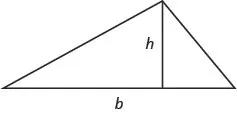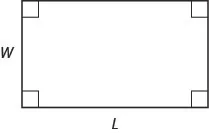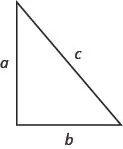Intermediate Algebra

# Key Concepts

Intermediate AlgebraKey Concepts

### Key Concepts

#### 9.1Solve Quadratic Equations Using the Square Root Property

• Square Root Property
• If $x2=kx2=k$, then $x=korx=−kx=korx=−k$ or $x=±kx=±k$

How to solve a quadratic equation using the square root property.
1. Step 1. Isolate the quadratic term and make its coefficient one.
2. Step 2. Use Square Root Property.
3. Step 3. Simplify the radical.
4. Step 4. Check the solutions.

#### 9.2Solve Quadratic Equations by Completing the Square

• Binomial Squares Pattern
If a and b are real numbers,• How to Complete a Square
1. Step 1. Identify b, the coefficient of x.
2. Step 2. Find $(12b)2,(12b)2,$ the number to complete the square.
3. Step 3. Add the $(12b)2(12b)2$ to x2 + bx
4. Step 4. Rewrite the trinomial as a binomial square
• How to solve a quadratic equation of the form ax2 + bx + c = 0 by completing the square.
1. Step 1. Divide by a to make the coefficient of x2 term 1.
2. Step 2. Isolate the variable terms on one side and the constant terms on the other.
3. Step 3. Find $(12·b)2,(12·b)2,$ the number needed to complete the square. Add it to both sides of the equation.
4. Step 4. Factor the perfect square trinomial, writing it as a binomial squared on the left and simplify by adding the terms on the right.
5. Step 5. Use the Square Root Property.
6. Step 6. Simplify the radical and then solve the two resulting equations.
7. Step 7. Check the solutions.

• The solutions to a quadratic equation of the form ax2 + bx + c = 0, $a≠0a≠0$ are given by the formula:
$x=−b±b2−4ac2ax=−b±b2−4ac2a$
1. Step 1. Write the quadratic equation in standard form, ax2 + bx + c = 0. Identify the values of a, b, c.
2. Step 2. Write the Quadratic Formula. Then substitute in the values of a, b, c.
3. Step 3. Simplify.
4. Step 4. Check the solutions.
• Using the Discriminant, b2 − 4ac, to Determine the Number and Type of Solutions of a Quadratic Equation
• For a quadratic equation of the form ax2 + bx + c = 0, $a≠0,a≠0,$
• If b2 − 4ac > 0, the equation has 2 real solutions.
• if b2 − 4ac = 0, the equation has 1 real solution.
• if b2 − 4ac < 0, the equation has 2 complex solutions.
• Methods to Solve Quadratic Equations:
• Factoring
• Square Root Property
• Completing the Square
• How to identify the most appropriate method to solve a quadratic equation.
1. Step 1. Try Factoring first. If the quadratic factors easily, this method is very quick.
2. Step 2. Try the Square Root Property next. If the equation fits the form ax2 = k or a(xh)2 = k, it can easily be solved by using the Square Root Property.
3. Step 3. Use the Quadratic Formula. Any other quadratic equation is best solved by using the Quadratic Formula.

• How to solve equations in quadratic form.
1. Step 1. Identify a substitution that will put the equation in quadratic form.
2. Step 2. Rewrite the equation with the substitution to put it in quadratic form.
3. Step 3. Solve the quadratic equation for u.
4. Step 4. Substitute the original variable back into the results, using the substitution.
5. Step 5. Solve for the original variable.
6. Step 6. Check the solutions.

#### 9.5Solve Applications of Quadratic Equations

• Methods to Solve Quadratic Equations
• Factoring
• Square Root Property
• Completing the Square
• How to use a Problem-Solving Strategy.
1. Step 1. Read the problem. Make sure all the words and ideas are understood.
2. Step 2. Identify what we are looking for.
3. Step 3. Name what we are looking for. Choose a variable to represent that quantity.
4. Step 4. Translate into an equation. It may be helpful to restate the problem in one sentence with all the important information. Then, translate the English sentence into an algebra equation.
5. Step 5. Solve the equation using good algebra techniques.
6. Step 6. Check the answer in the problem and make sure it makes sense.
7. Step 7. Answer the question with a complete sentence.
• Area of a Triangle
• For a triangle with base, b, and height, h, the area, A, is given by the formula $A=12bh.A=12bh.$• Area of a Rectangle
• For a rectangle with length, L, and width, W, the area, A, is given by the formula A = LW.• Pythagorean Theorem
• In any right triangle, where a and b are the lengths of the legs, and c is the length of the hypotenuse, a2 + b2 = c2.• Projectile motion
• The height in feet, h, of an object shot upwards into the air with initial velocity, v0, after t seconds is given by the formula h = −16t2 + v0t.

#### 9.6Graph Quadratic Functions Using Properties

• Parabola Orientation
• For the graph of the quadratic function $f(x)=ax2+bx+c,f(x)=ax2+bx+c,$ if
• a > 0, the parabola opens upward.
• a < 0, the parabola opens downward.
• Axis of Symmetry and Vertex of a Parabola The graph of the function $f(x)=ax2+bx+cf(x)=ax2+bx+c$ is a parabola where:
• the axis of symmetry is the vertical line $x=−b2a.x=−b2a.$
• the vertex is a point on the axis of symmetry, so its x-coordinate is $−b2a.−b2a.$
• the y-coordinate of the vertex is found by substituting $x=−b2ax=−b2a$ into the quadratic equation.
• Find the Intercepts of a Parabola
• To find the intercepts of a parabola whose function is $f(x)=ax2+bx+c:f(x)=ax2+bx+c:$
$y-interceptx-interceptsLetx=0and solve forf(x).Letf(x)=0and solve forx.y-interceptx-interceptsLetx=0and solve forf(x).Letf(x)=0and solve forx.$
• How to graph a quadratic function using properties.
1. Step 1. Determine whether the parabola opens upward or downward.
2. Step 2. Find the equation of the axis of symmetry.
3. Step 3. Find the vertex.
4. Step 4. Find the y-intercept. Find the point symmetric to the y-intercept across the axis of symmetry.
5. Step 5. Find the x-intercepts. Find additional points if needed.
6. Step 6. Graph the parabola.
• Minimum or Maximum Values of a Quadratic Equation
• The y-coordinate of the vertex of the graph of a quadratic equation is the
• minimum value of the quadratic equation if the parabola opens upward.
• maximum value of the quadratic equation if the parabola opens downward.

#### 9.7Graph Quadratic Functions Using Transformations

• Graph a Quadratic Function of the form $f(x)=x2+kf(x)=x2+k$ Using a Vertical Shift
• The graph of $f(x)=x2+kf(x)=x2+k$ shifts the graph of $f(x)=x2f(x)=x2$ vertically k units.
• If k > 0, shift the parabola vertically up k units.
• If k < 0, shift the parabola vertically down $|k||k|$ units.
• Graph a Quadratic Function of the form $f(x)=(x−h)2f(x)=(x−h)2$ Using a Horizontal Shift
• The graph of $f(x)=(x−h)2f(x)=(x−h)2$ shifts the graph of $f(x)=x2f(x)=x2$ horizontally h units.
• If h > 0, shift the parabola horizontally left h units.
• If h < 0, shift the parabola horizontally right $|h||h|$ units.
• Graph of a Quadratic Function of the form $f(x)=ax2f(x)=ax2$
• The coefficient a in the function $f(x)=ax2f(x)=ax2$ affects the graph of $f(x)=x2f(x)=x2$ by stretching or compressing it.
If $0<|a|<1,0<|a|<1,$ then the graph of $f(x)=ax2f(x)=ax2$ will be “wider” than the graph of $f(x)=x2.f(x)=x2.$
If $|a|>1,|a|>1,$ then the graph of $f(x)=ax2f(x)=ax2$ will be “skinnier” than the graph of $f(x)=x2.f(x)=x2.$
• How to graph a quadratic function using transformations
1. Step 1. Rewrite the function in $f(x)=a(x−h)2+kf(x)=a(x−h)2+k$ form by completing the square.
2. Step 2. Graph the function using transformations.
• Graph a quadratic function in the vertex form $f(x)=a(x−h)2+kf(x)=a(x−h)2+k$ using properties
1. Step 1. Rewrite the function in $f(x)=a(x−h)2+kf(x)=a(x−h)2+k$ form.
2. Step 2. Determine whether the parabola opens upward, a > 0, or downward, a < 0.
3. Step 3. Find the axis of symmetry, x = h.
4. Step 4. Find the vertex, (h, k).
5. Step 5. Find they-intercept. Find the point symmetric to the y-intercept across the axis of symmetry.
6. Step 6. Find the x-intercepts, if possible.
7. Step 7. Graph the parabola.

• Solve a Quadratic Inequality Graphically
1. Step 1. Write the quadratic inequality in standard form.
2. Step 2. Graph the function $f(x)=ax2+bx+cf(x)=ax2+bx+c$ using properties or transformations.
3. Step 3. Determine the solution from the graph.
• How to Solve a Quadratic Inequality Algebraically
1. Step 1. Write the quadratic inequality in standard form.
2. Step 2. Determine the critical points -- the solutions to the related quadratic equation.
3. Step 3. Use the critical points to divide the number line into intervals.
4. Step 4. Above the number line show the sign of each quadratic expression using test points from each interval substituted into the original inequality.
5. Step 5. Determine the intervals where the inequality is correct. Write the solution in interval notation.
Order a print copy

As an Amazon Associate we earn from qualifying purchases.